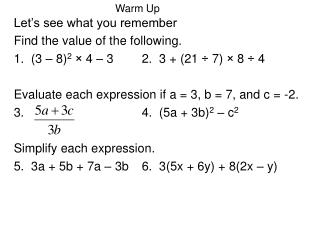DownloadDownload PresentationLet’s see what you remember Find the value of the following.

# Let’s see what you remember Find the value of the following.

Download Presentation## Let’s see what you remember Find the value of the following.

- - - - - - - - - - - - - - - - - - - - - - - - - - - E N D - - - - - - - - - - - - - - - - - - - - - - - - - - -
##### Presentation Transcript

1. Warm Up Let’s see what you remember Find the value of the following. 1. (3 – 8)2 × 4 – 3 2. 3 + (21 ÷ 7) × 8 ÷ 4 Evaluate each expression if a = 3, b = 7, and c = -2. 3. 4. (5a + 3b)2 – c2 Simplify each expression. 5. 3a + 5b + 7a – 3b 6. 3(5x + 6y) + 8(2x – y)

2. 9 97 Find the value of the following. 1. (3 – 8)2 × 4 – 3 2. 3 + (21 ÷ 7) × 8 ÷ 4 Evaluate each expression if a = 3, b = 7, and c = -2. 3. 4. (5a + 3b)2 – c2 Simplify each expression. 5. 3a + 5b + 7a – 3b 6. 3(5x + 6y) + 8(2x – y) 1292 31x + 10y 10a + 2b

3. Paper Cup Analysis Exploration 1-1a in small groups (15-20 minutes) • Issue textbooks while students complete explorations • Issue course outline: State rules and expectations (10 minutes) • Students will read pp. 1-5 after they finish the exploration • PPT to match pp 1-5 in the text (10 minutes) • Exploration 1-2a Names of Functions in small group as a review of linear, quadratic, power, exponential, inverse, quartic, and rational functions (20 minutes) • Summary of exploration and vocabulary (15 mins) • Assignment pg. 5 #’s 1-6 and finish exploration 1-2a Math 4 day 1 agenda

4. Standard(s): MA3A4. Students will investigate functions. a. Compare and Contrast properties of functions within and across the following types: linear, quadratic, polynomial, power, rational, exponential, logarithmic, trigonometric, and piecewise. Essential Question(s): How can I represent functions algebraically, graphically, numerically and verbally?

5. Exploration 1-1a Summary Have one member from each group sketch their answer for #2 on the board and then write the groups answer for #5 on the board. Be prepared to discuss this exploration. This section in your textbook reviews functions graphically, numerically, and algebraically. It begins by presenting a graph and equation for the relationship between coffee temperature and time. After you read pp. 1-5 be prepared to discuss the vocabulary: Function, Mathematical Model, Dependent Variable, Independent Variable, Domain, Range, Asymptote, Extrapolation, and Interpolation.

6. Relationship between coffee temperature and time Graphically Numerically Verbally Algebraically When you pour a cup of coffee it begins at a temperature of 90 degrees Celsius, it cools more rapidly at first, then less rapidly, finally approaching the room temperature of 20 degrees Celsius.

7. Discuss/define: • Function • Mathematical Model • Dependent Variable • Independent Variable • Domain • Range, • Asymptote • Extrapolation • Interpolation 1.2 Types of functions Note- the notation f(x) represent substitution not multiplication. A relation is a function when each value in the domain there is only one corresponding value in the domain. Pay close attention to the difference between an exponential and a power function

8. Complete Exploration 1.2aNames of FunctionsGraphing Calculators Allowed Time allowed 15-20 minutesBegin CW/HW if you finish early • Assignment pg. 5 #’s 1-6.

9. SummaryEach group is assigned one problem to describe to the class Direct variation - special case of a linear function with y-intercept 0, power function with a power of 1, and polynomial function with only a linear term. In other words its graph is a straight line through the origin. Can you think of any from science? Hooke’s law y=kx or Newton’s second law of motion F=ma An Inverse variation function is a power function with a negative exponent , and asymptotes at each axis. Can you think of any from science? Boyle’s law P= k/V

10. Restricted Domains and Boolean Variables A Boolean variable is a variable that has a given condition attached to it. If the condition is true, the variable equals 1, if the condition is false the variable equals 0. To plot a function is a restricted domain, divide by any one term of the function’s equation by the appropriate Boolean variable. If you write a function where the dominator is the restriction you will only get the graph for the values where it is true and the restriction yields a 1, when it is not true it will yield a 0 and division by zeros is undefined so there will be no graph when the restriction is not true. Example 1, in your book p. 11 will only graph for the values when 3< x< 10 if you let y1 = 3x + 26 / (x > 3 and x < 10) Note: the inequality signs are found under 2nd MATH Test #4 and #6 and the word “and” is found under 2nd MATH arrow over to logic #1, try graphing this. Make sure your window for the y values will show the answer 56 • Assignment pg. 5 #’s 1-6 and finish exploration 1-2a